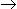# Chemical Engineering - Chemical Reaction Engineering

### Exercise :: Chemical Reaction Engineering - Section 10

1.

The conversion for a first order liquid phase reaction. AB in a CSTR is 50%. If another CSTR of the same volume is connected in series, then the % conversion at the exit of the second reactor will be

 A. 60 B. 75 C. 90 D. 100

Explanation:

No answer description available for this question. Let us discuss.

2.

What is the dispersion number for a CSTR?

 A. 0 B. 1 C. < 1 D. ∞

Explanation:

No answer description available for this question. Let us discuss.

3.

Rate of a chemical reaction is independent of the concentration of the reactants for a __________ reaction.

 A. zero order B. third order C. consecutive D. none of these

Explanation:

No answer description available for this question. Let us discuss.

4.

With increase in temperature, the rate constant obeying Arhenious equation

 A. increases. B. decreases. C. decreases exponentially. D. can either increase or decrease ; depends on the frequency factor.

Explanation:

No answer description available for this question. Let us discuss.

5.

Bulk diffusion in catalyst pore __________ with increase in pressure.

 A. increases B. decreases C. remains unchanged D. increases exponentially